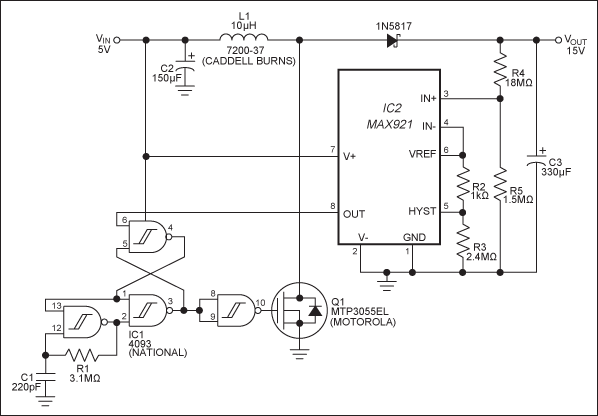# Boost Converter Has High Efficiency at Light Loads

### Abstract

This design note shows how a boost converter is designed from a quad Schmitt-trigger NAND gate and the MAX921, a comparator with integrated voltage reference. Consuming only microwatts of power, this 5V-to-15V boost converter provides low load currents with high efficiency. The efficiency is > 90% for load currents between 1mA and 8mA.

In most DC-DC converters, the normal supply currents do not allow high efficiency at low load currents. The circuit in Figure 1, however, contains micropower components that enable it to maintain 90% efficiency for load currents as low as 1mA. IC1 (a quad Schmitt-trigger NAND gate) draws maximum quiescent currents of only 0.25µA, and IC2 (a combination voltage reference and comparator) draws only 2.5µA.IC2 compares its own reference voltage against the circuit output, VOUT. The resulting comparator output (pin 8) is high when VOUT is above its threshold and low otherwise. The quad NAND gate is configured as an oscillator, a set/reset latch, and a buffer inverter. The latch blocks oscillator pulses when the comparator output is high. When it goes low, the pulses pass through to Q1's gate and activate the boost regulator.

R4 and R5 help determine the circuit's DC output level: VOUT = VREF(1 + R4/R5). The output voltage ripple for light loads depends on the comparator's hysteresis. With R3 = 2.4MΩ, the hysteresis in millivolts equals the value of R2 in kilohms. Then, the ripple in millivolts equals VREF(1 + R4/R5)(R2), where R2 is in kilohms. For this circuit, ripple = 1.182V(1 + 18/1.5)(1) = 15.4mV.